How Cheenta works to ensure student success?
Explore the Back-Story

# Multiplicative group from fields: TIFR GS 2018 Part A Problem 17# Understand the problem

The multiplicative group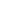is isomorphic to a subgroup of the multiplicative group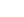Hint 1
We will write them as (Z/7Z)* and (Z/31Z)* respectively instead of the notations used.
• Observe that (Z/7Z)* has order 6 and (Z/31Z)* has order 30.So there is a possibility that (Z/7Z)* is a subgroup of (Z/31Z)* by Lagrange’s Theorem.
• So we need to go into the structure of the groups to solve this problem.Hence we proceed!
• Let us investigate the group (Z/7Z)*.It consists of {1,2,3,4,5,6 mod 7}.Observe that 3 mod 7 generates the group.
• So naturally the next question is that whether (Z/31Z)* rather is there any general result?
Hint 2
• In fact the following theorem is true and describes the cyclicity (Z/nZ)* to some extent.
• Theorem: If p is a prime then (Z/pZ)* is cyclic. (Check!) {Check the bonus question for the complete characterization of cyclicity of (Z/nZ)* done by Gauss.}
Hint 3
• So (Z/7Z)* and (Z/31Z)* are cyclic groups of order 6 and 30 respectively with generators say A and B respectively.
• Now take the element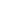.The following Lemma describes its order.
• Lemma: If g is the generator of the cyclic group of order n. Then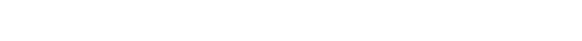has order 6 and hence it is isomorphic to (Z/7Z)*.
• Hence the answer is True.
Hint 4
Bonus Problem:
• Theorem: The group (Z/nZ)* is cyclic if and only if n is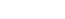or 2.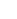, where p is an odd prime and k > 0. This was first proved by Gauss. (Wow!)
Solve and Salvage if Possible.

# Connected Program at Cheenta

The higher mathematics program caters to advanced college and university students. It is useful for I.S.I. M.Math Entrance, GRE Math Subject Test, TIFR Ph.D. Entrance, I.I.T. JAM. The program is problem driven. We work with candidates who have a deep love for mathematics. This program is also useful for adults continuing who wish to rediscover the world of mathematics.

# Understand the problem

The multiplicative groupis isomorphic to a subgroup of the multiplicative groupHint 1
We will write them as (Z/7Z)* and (Z/31Z)* respectively instead of the notations used.
• Observe that (Z/7Z)* has order 6 and (Z/31Z)* has order 30.So there is a possibility that (Z/7Z)* is a subgroup of (Z/31Z)* by Lagrange’s Theorem.
• So we need to go into the structure of the groups to solve this problem.Hence we proceed!
• Let us investigate the group (Z/7Z)*.It consists of {1,2,3,4,5,6 mod 7}.Observe that 3 mod 7 generates the group.
• So naturally the next question is that whether (Z/31Z)* rather is there any general result?
Hint 2
• In fact the following theorem is true and describes the cyclicity (Z/nZ)* to some extent.
• Theorem: If p is a prime then (Z/pZ)* is cyclic. (Check!) {Check the bonus question for the complete characterization of cyclicity of (Z/nZ)* done by Gauss.}
Hint 3
• So (Z/7Z)* and (Z/31Z)* are cyclic groups of order 6 and 30 respectively with generators say A and B respectively.
• Now take the element.The following Lemma describes its order.
• Lemma: If g is the generator of the cyclic group of order n. Thenhas order 6 and hence it is isomorphic to (Z/7Z)*.
• Hence the answer is True.
Hint 4
Bonus Problem:
• Theorem: The group (Z/nZ)* is cyclic if and only if n isor 2., where p is an odd prime and k > 0. This was first proved by Gauss. (Wow!)
Solve and Salvage if Possible.

# Connected Program at Cheenta

The higher mathematics program caters to advanced college and university students. It is useful for I.S.I. M.Math Entrance, GRE Math Subject Test, TIFR Ph.D. Entrance, I.I.T. JAM. The program is problem driven. We work with candidates who have a deep love for mathematics. This program is also useful for adults continuing who wish to rediscover the world of mathematics.

# Similar Problems

This site uses Akismet to reduce spam. Learn how your comment data is processed.

### 2 comments on “Multiplicative group from fields: TIFR GS 2018 Part A Problem 17”

1.Amritha says:

Very useful..... Good metod.... Keep on going

1.Ashani Dasgupta says:

### Knowledge Partner